## South Green tutorials pages

Description A step by step guide to start on R
Authors christine Tranchant-Dubreuil (christine.tranchant@ird.fr)
Creation Date 21/09/2018

### Displaying and manipulating the dataframe content

##### Printing a column - `dataframe[colNum|colName]` or `dataframe\$colName`
• output result (line)
• output result (column)

Line 2

##### Printing one line, one column - `dataframe[lineNum,colNum]`

Line 2, column 6 then line 2, column 3

##### Printing one column of some lines - `dataframe[c(line1,line2,line3),colNum]`

Print the complete lines 1, 3, 7, 6 then just the column 3 of the same lines

### Manipulating the content of a dataframe

##### Adding a new column
• A new collumn firstly filled with “NA”
• The new column receives the result of an operation

##### Extracting one part of a dataframe into a new dataframe - `subset(dataframe)`
• Extract only lines about chromosome (Organism column equals to “dna:chromosome”) - `==`
• Extract only lines about scaffold (Organism column not equals to “dna:chromosome”) - `!=`

### Plotting data from a dataframe with `ggplot` library

To build a plot, the same following basic template can be used for several plot types :

`ggplot(data = <DATA>, aes = (<MAPPINGS>)) + <GEOM_FUNCTION>()`

• use the ggplot() function and bind the plot to a specific data frame using the `data` argument
• use the `aes` function to select the variables to be plotted and to define how tu present them in the graph (e.g. x/y positions, size, shape, color)
• add ‘geoms’ – graphical representations of the data in the plot (points, lines, bars) such as `geom_point()`, `geom_boxplot()`,`geom_line()`, `geom-bar()`

### with `ggplot()` + `geom_bar(stat = "identity")`]

ggplot is a plotting package that makes simple to create complex plots from data stored in a data frame. It provides a programmatic interface for specifying what variables to plot, how they are displayed, and general visual properties.

https://datacarpentry.org/r-socialsci/04-ggplot2/

##### Creating a barplot with `geom_bar(stat = "identity")`]

Barplots are useful for visualizing categorical data. By default, geom_bar accepts a variable for x, and plots the number of instances each value of x (in this case, chromosome) appears in the dataset.

Here, we will :

• plot the size (mb) of each chromosome from each genome version with geom_bar
• use the fill aesthetic for the geom_bar() geom to color bars by the size of each chromosome for each genome version
• By default, geom_bar uses stat=”bin” to make the height of each bar equal to the number of cases in each group (it is incompatible with mapping values to the y aesthetic). Here we use `stat="identity"` because we want the heights of the bars to represent values in the data (with a y aesthetic).

http://www.sthda.com/french/wiki/ggplot2-barplots-guide-de-demarrage-rapide-logiciel-r-et-visualisation-de-donnees#barplots-basiques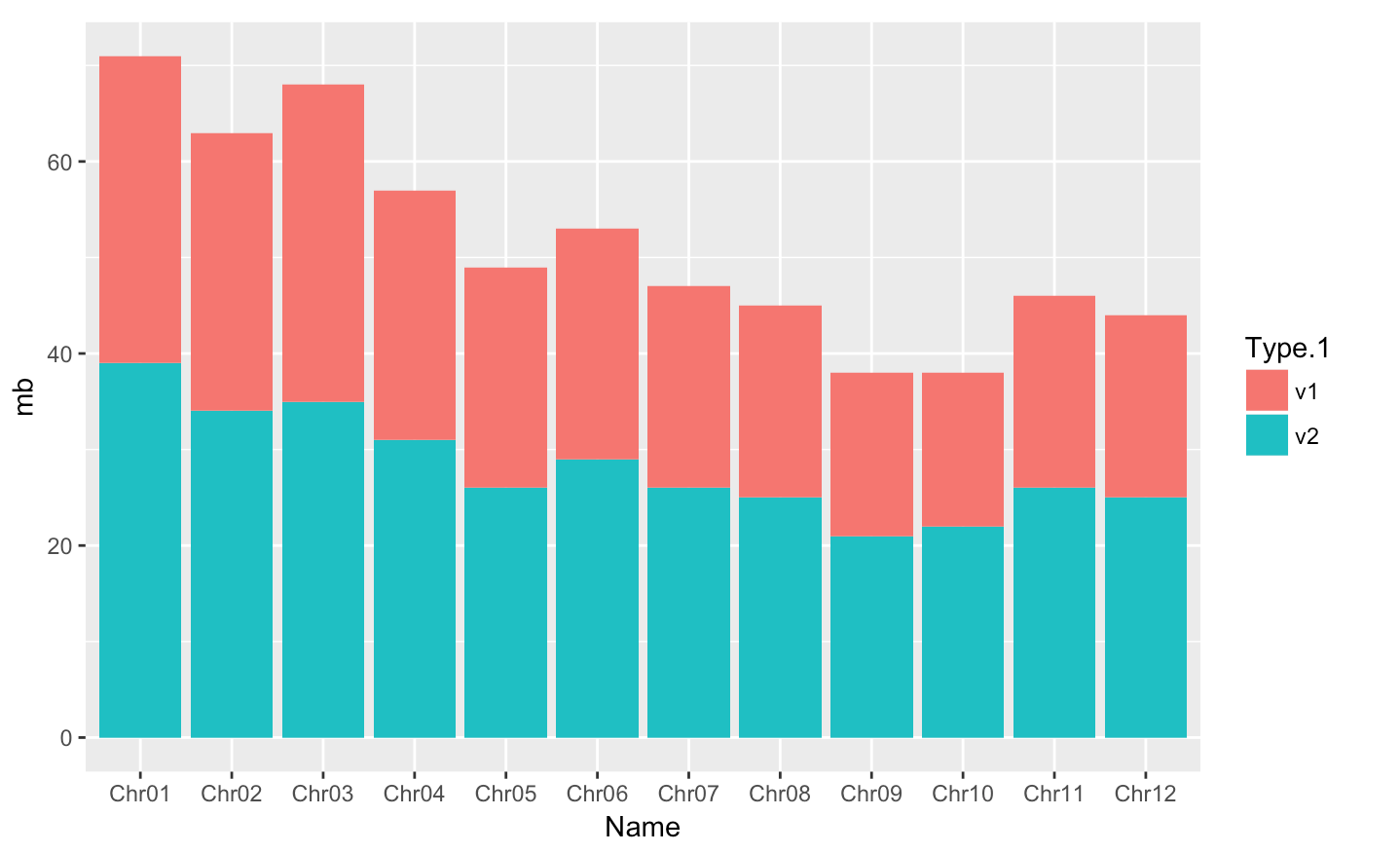##### distinct plot - `position=position_dodge()` and flip plot with `coord_flip()`
• We can separate the portions of the stacked bar that correspond to each genome version and put them side-by-side by using the position argument for geom_bar() and setting it to “dodge”.

• `coord_flip()`: Flip cartesian coordinates so that horizontal becomes vertical, and vertical, horizontal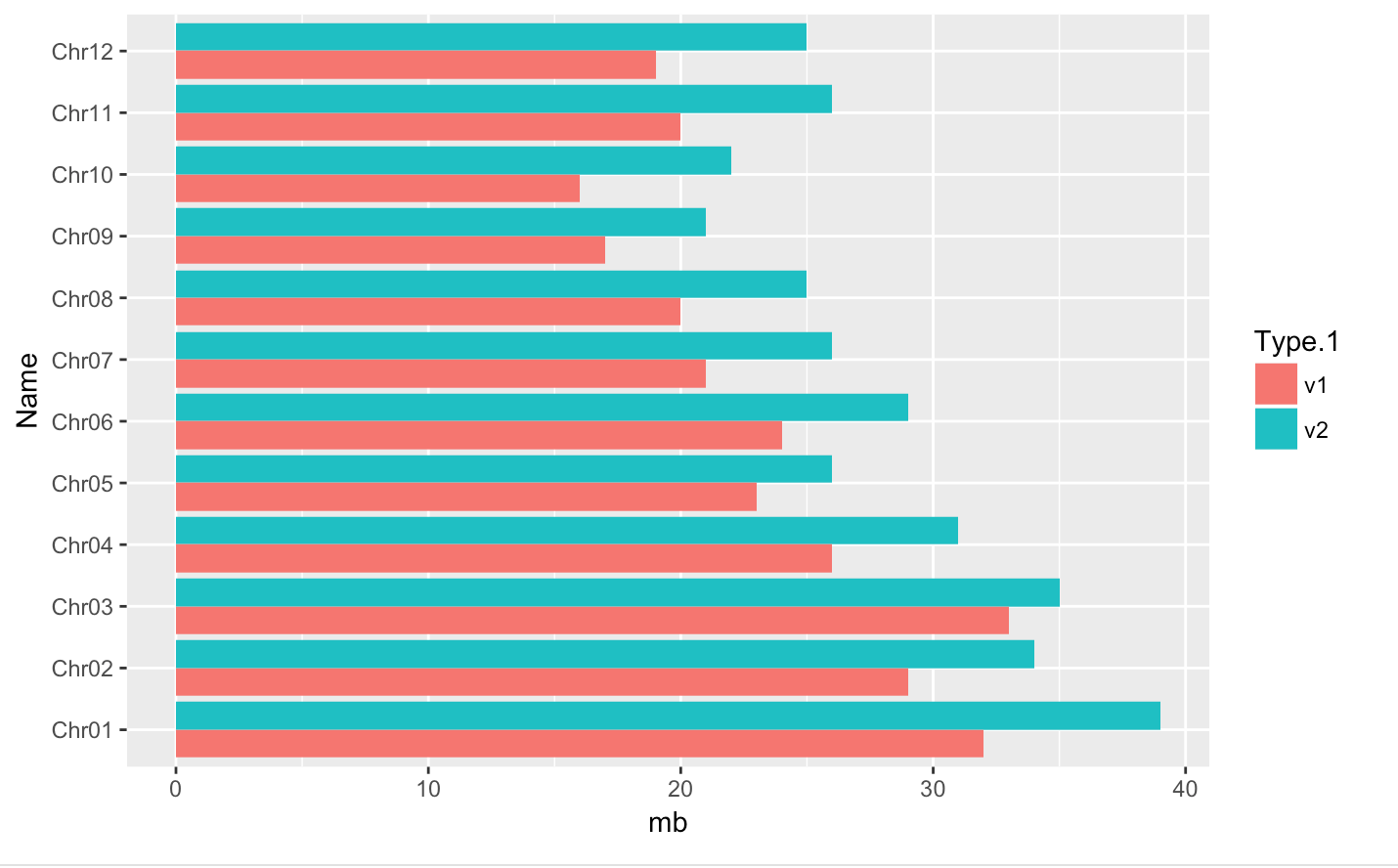##### Creating a barplot with `geom_bar(stat = "count")`]
• we use `stat="count"` to make the height of each bar equal to the count of lines
• Adding Labels and Titles with `ylab(<Y-LABEL>)`, `xlab(<X-LABEL>)` , `ggtitle(<TITLE-LABEL>)`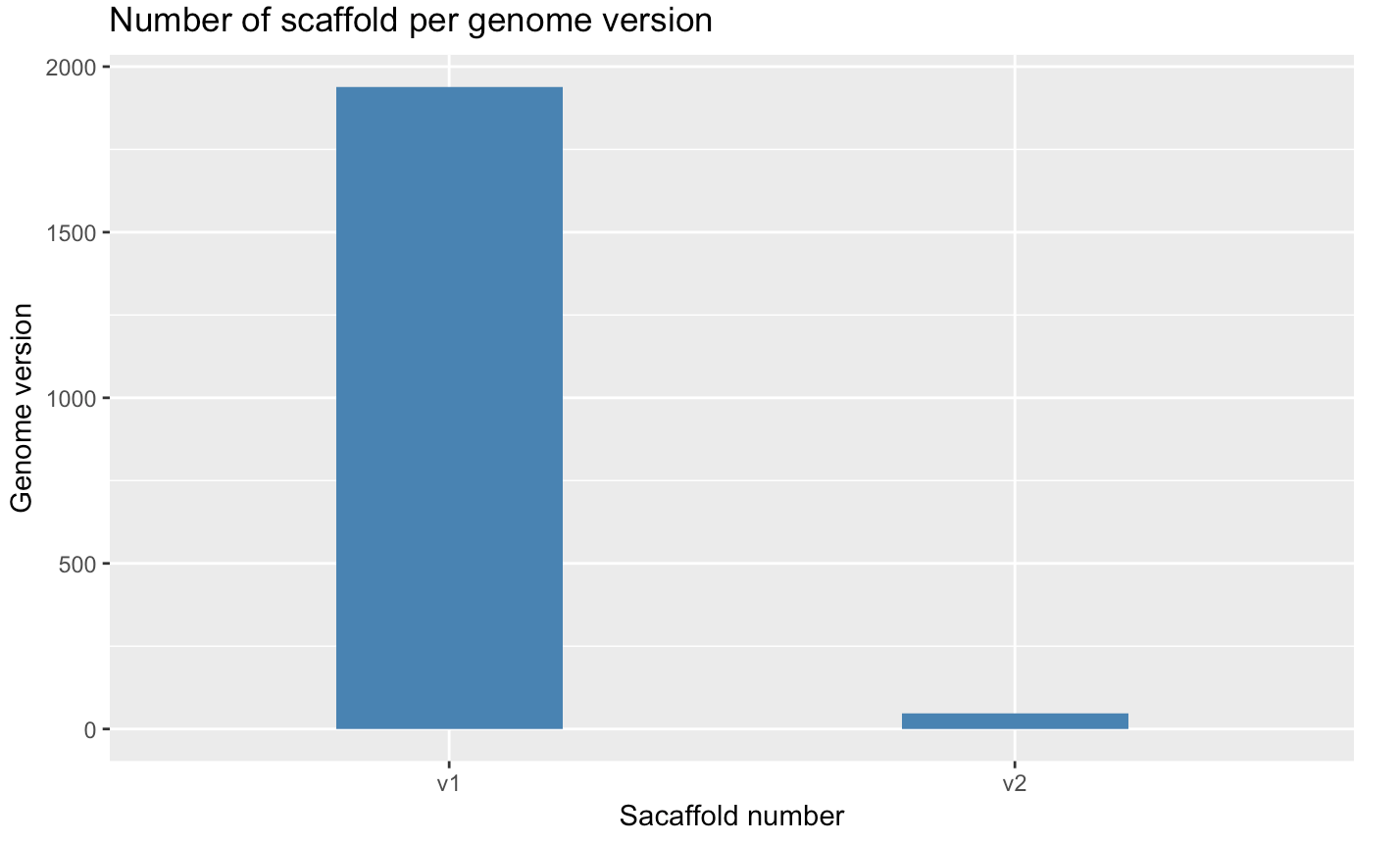##### Plotting a violin plot - `geom_violin()`
• Create a violin plot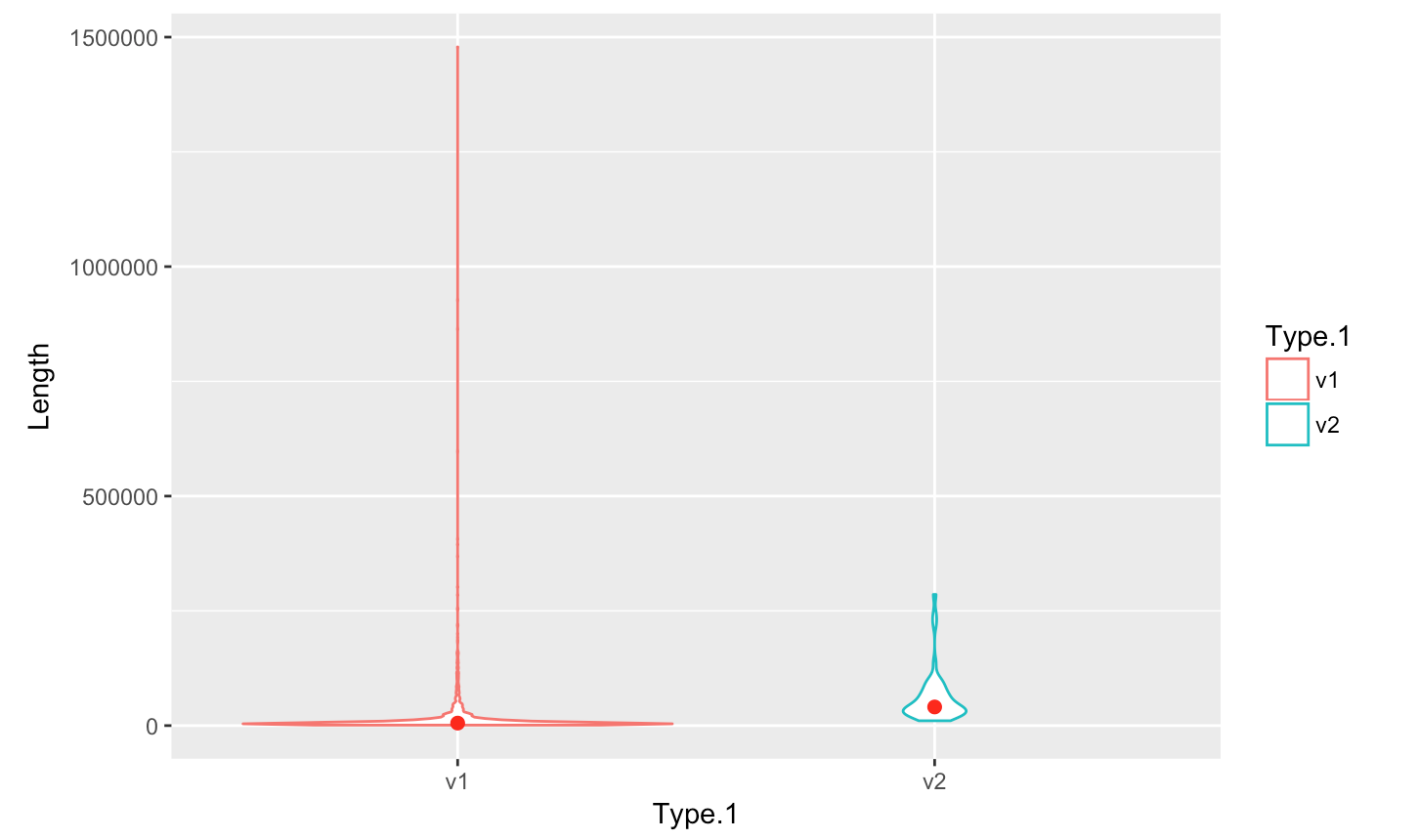• Save plot into a file

##### Arranging plots in a grid - `grid.arrange()`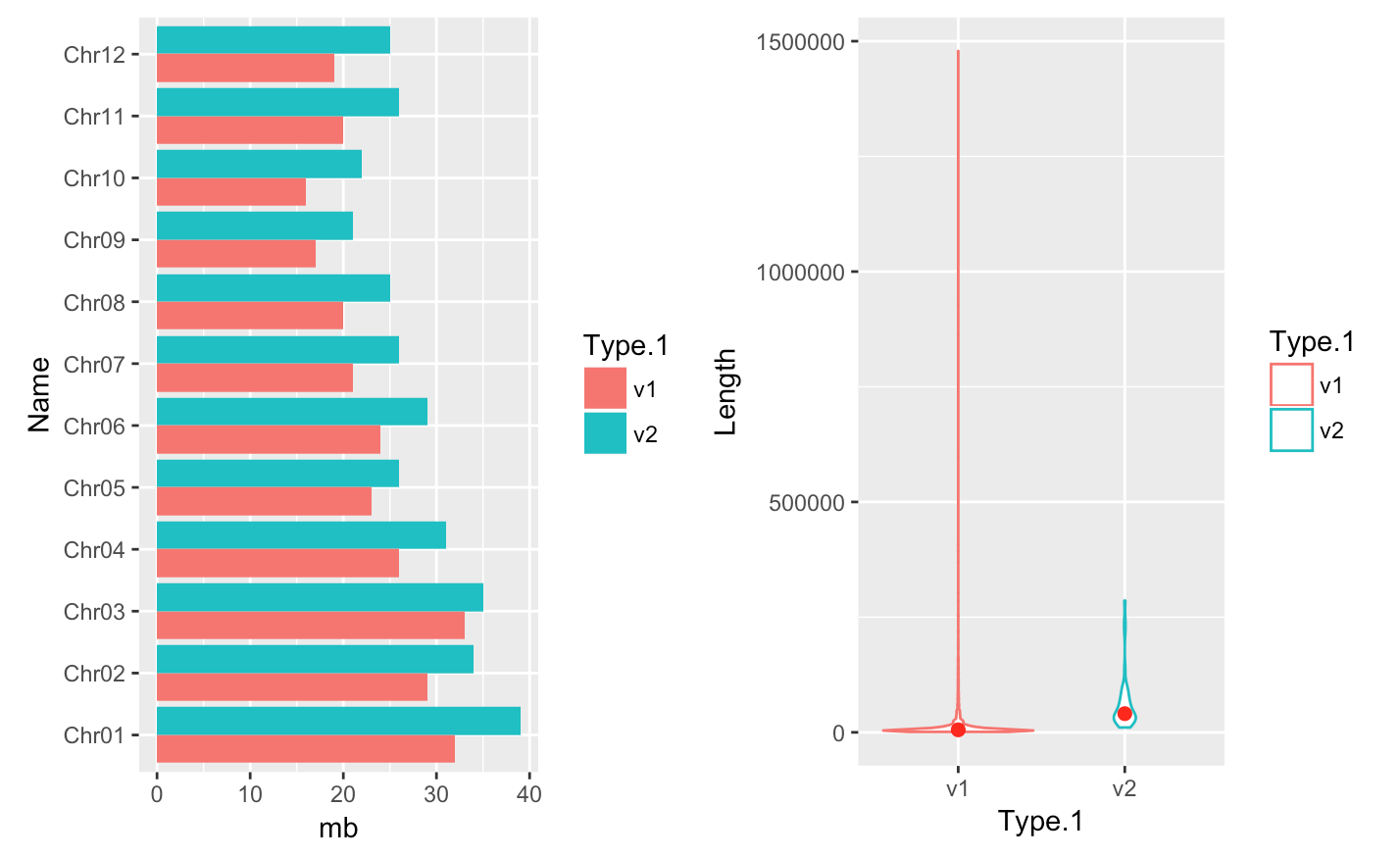### Displaying and manipulating the dataframe content

##### Printing the first lines - `head(dataframe)`